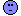## Nervous ticks in C - a reminder of the cruel logic of logic [Software]

Hi all,

sometimes logic totally sucks.

I am making code in C for plotting various graphs, and am making a little routine for inserting ticks on an axis. Ticks have to be "nice" (yes, that's also the word used in the R documentation).

So, let us say the maximum observed value is x=0.234567 and I want ticks evenly dispersed at a distance of dx=0.05 (say from 0.0 and upwards). So, I thought I was clever and calculated the "maximum" tick as
xmax = dx*ceil(x/dx)

You can see one definition of the ceil function here.

So, one way to test it is like this, which will print the actual ticks:
 int Test10001(double x, double dx) {     double xmax;     xmax=dx*ceil(x/dx);     x=0.0;     while (x<=xmax)     {         printf("x=%f\n",x);         x=x+dx;     }     return(0); }

When I call that function with arguments 0.234567 and 0.05 it prints:
x=0.000000 x=0.050000 x=0.100000 x=0.150000 x=0.200000

but it doesn't print 0.25 ?!???If I add a line like
printf("xmax=%f\n", xmax);

then I get
xmax=0.250000

This is the beauty of C. Sometimes it is all-out warfare with this language and little tasks that should take 2 minutes take 2 days and end up with theory around little endians and data types and ANSI specifications and God knows what else.

Pass or fail!
ElMaestroIng. Helmut Schütz